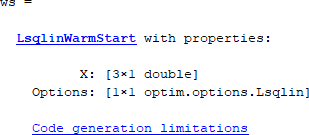# optimwarmstart

Create warm start object

## Syntax

``ws = optimwarmstart(x0,options)``
``ws = optimwarmstart(x0,options,Name,Value)``

## Description

example

````ws = optimwarmstart(x0,options)` creates a warm start object `ws` for use with the solver indicated in `options`. For an example using a warm start object, see Warm Start quadprog.```

example

````ws = optimwarmstart(x0,options,Name,Value)` incorporates memory bounds in `ws` using name-value arguments. Use memory bounds only when generating code.```

## Examples

collapse all

Create a default warm start object for `quadprog`.

```x0 = [1 3 5]; options = optimoptions('quadprog','Algorithm','active-set'); ws = optimwarmstart(x0,options)```
```ws = QuadprogWarmStart with properties: X: [3×1 double] Options: [1×1 optim.options.Quadprog] Code generation limitations```

Create an `lsqlin` warm start object for code generation with memory limits.

```x0 = [1 3 5]; options = optimoptions('lsqlin','Algorithm','active-set'); ws = optimwarmstart(x0,options,... 'MaxLinearEqualities',30,... 'MaxLinearInequalities',5)```Click the `Code generation limitations` link to see the memory settings.

``` MaxLinearEqualities: 30 MaxLinearInequalities: 5 ```

## Input Arguments

collapse all

Initial point, specified as a real array. This point is stored in `ws.X`.

Example: `10*rand(5,1)`

Data Types: `double`

Optimization options, specified as the output of `optimoptions`. You must specify at least a supported solver, either `lsqlin` or `quadprog`, and `'active-set'` for the and `Algorithm` option. For example, enter the following code to specify the `quadprog` solver.

`options = optimoptions('quadprog','Algorithm','active-set');`

These options are stored in `ws.Options`.

### Name-Value Arguments

Specify optional pairs of arguments as `Name1=Value1,...,NameN=ValueN`, where `Name` is the argument name and `Value` is the corresponding value. Name-value arguments must appear after other arguments, but the order of the pairs does not matter.

Before R2021a, use commas to separate each name and value, and enclose `Name` in quotes.

Example: ```ws = optimwarmstart(x0,options,'MaxLinearEqualities',30,'MaxLinearInequalities',5)``` specifies up to 30 linear equalities and 5 linear inequalities.

Maximum number of linear equality constraints, specified as a positive integer. To allocate enough memory for equality constraints, specify the maximum number of equality constraints during the entire run of the code.

Use this argument only for code generation without dynamic memory allocation. You must use both this argument and `'MaxLinearInequalities'`.

The value of this argument is stored in `ws.MaxLinearEqualities`.

Example: `25`

Data Types: `double`

Maximum number of linear inequality constraints, specified as a positive integer. To allocate enough memory for inequality constraints, specify the maximum number of inequality constraints during the entire run of the code.

Use this argument only for code generation without dynamic memory allocation. You must use both this argument and `'MaxLinearEqualities'`.

The value of this argument is stored in `ws.MaxLinearInequalities`.

Example: `25`

Data Types: `double`

## Output Arguments

collapse all

Warm start object, returned as an `LsqlinWarmStart` object or a `QuadprogWarmStart` object. For an example using a warm start object, see Warm Start quadprog.

`ws` has the following read-only properties:

• `X` — Initial point

• `Options` — Optimization options

• `MaxLinearEqualities` — Maximum number of linear equalities for code generation

• `MaxLinearInequalities` — Maximum number of linear inequalities for code generation

To change any properties of `ws`, recreate the object by calling `optimwarmstart`.

## Algorithms

A warm start object maintains a list of active constraints from the previous solved problem. The solver carries over as much active constraint information as possible to solve the current problem. If the previous problem is too different from the current one, no active set information is reused. In this case, the solver effectively executes a cold start in order to rebuild the list of active constraints.

## Version History

Introduced in R2021a